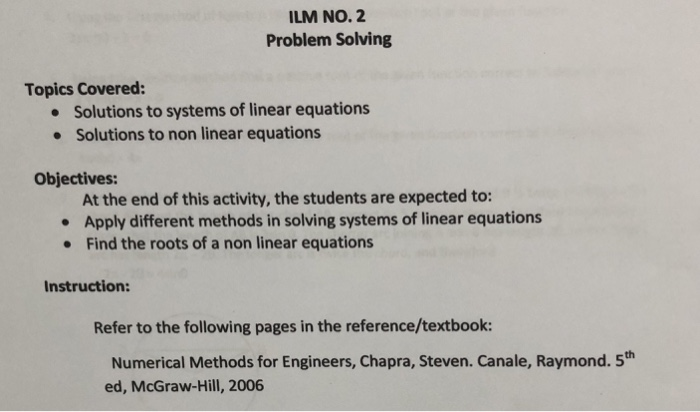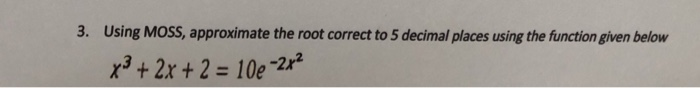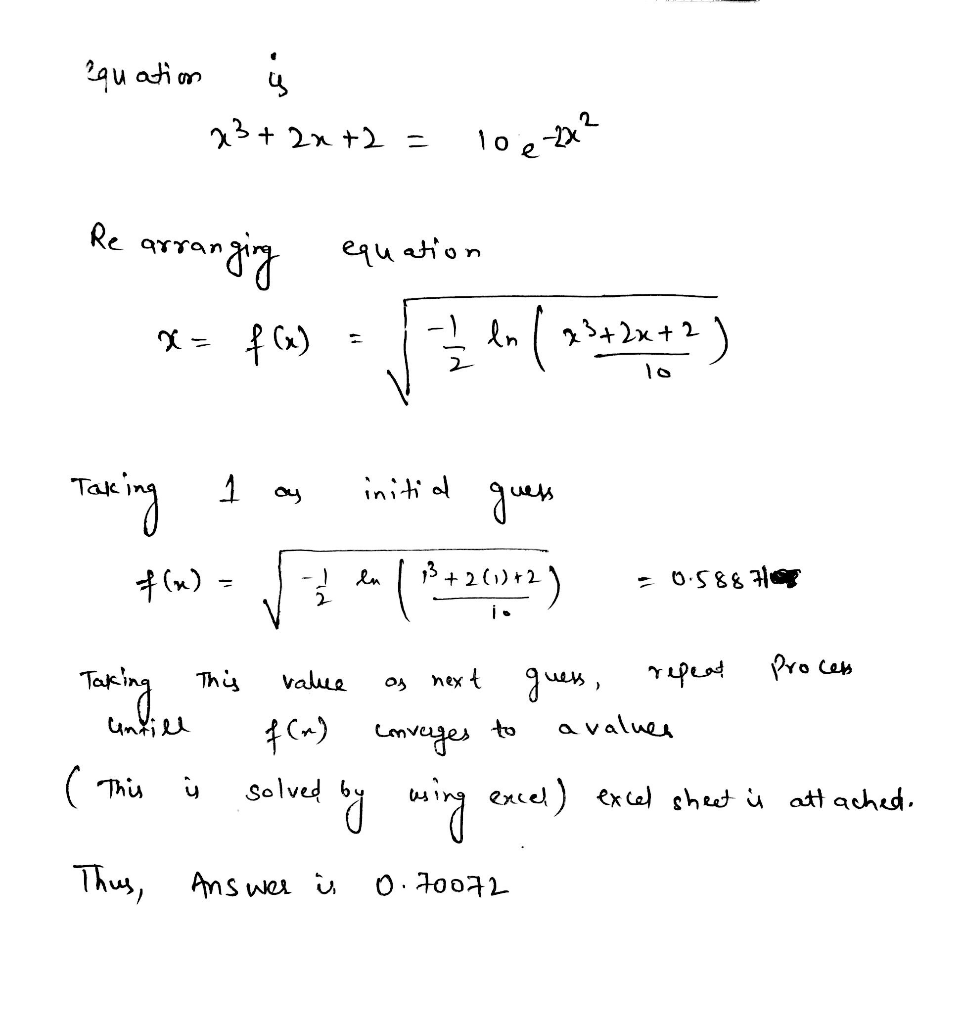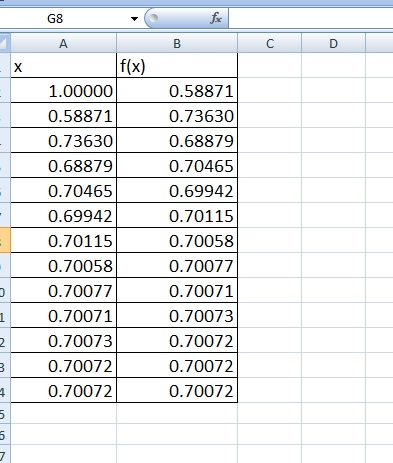# ILM NO. 2 Problem Solving Topics Covered: Solutions to systems of linear equations Solutions to n...

i want solve part 3
useing mathood of successive substitution (moss)ILM NO. 2 Problem Solving Topics Covered: Solutions to systems of linear equations Solutions to non linear equations Objectives: At the end of this activity, the students are expected to: Apply different methods in solving systems of linear equations Find the roots of a non linear equations Instruction: Refer to the following pages in the reference/textbook: Numerical Methods for Engineers, Chapra, Steven. Canale, Raymond. 5th ed, McGraw-Hill, 2006
3. Using MOss, approximate the root correct to 5 decimal places using the function given below x3+ 2x +2- 10e-22 -2x2##### Add Answer of: ILM NO. 2 Problem Solving Topics Covered: Solutions to systems of linear equations Solutions to n...
More Homework Help Questions Additional questions in this topic.

• #### A project needs to be created for the topic - Consumer Awareness with the topics to be covered be :-1)Consumer Awareness and its needs

Need Online Homework Help?